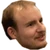## FANDOM

2,419 Pages

Spending a power counter from IT Department creates a constant ability that checks how many power counters are left on IT Department each time a power counter is spent. If you spend multiple power counters (either to boost the strength of a single piece of ice multiple times or to boost the strength of multiple pieces of ice), then each time a power counter is spent IT Department "updates" the boosted strength according to how many power counters are left on IT Department as of the last spent power counter.

## ControversyEdit

On December 29, 2014, Steve Lyman asked Lukas on Twitter how IT Department is supposed to work. The first answer Lukas gave was incorrect and caused a large uproar on Twitter as people struggled to understand. but due to the contradiction with the first tweet it was lost in the sea of tweets.

The following day, Lukas apologized and gave a more direct and correct response on how IT Department is intended to work. See the rest of the Twitter thread to read more about the misunderstanding.

## Steve Lyman's QuestionEdit

3 counter IT Dept. Is it: -2 counters = +5 strength (3+2), or -2 counters = +3 strength (3 counters on ITD incl. those removed)?

### Lukas's Revised AnswerEditThere is reason to use IT Dept with 3 counters on an ice. 2 counters makes it +2 and +2. Only spending 3 gives +3.Also, if you have more than 3 counters on IT Dept you can still get more strength for one ice.

## Laury Plant's QuestionEdit

I'm still misunderstanding, how is this 18 and not 19? Rechecking past resolved events and modifying them in retrospect?

## Ben Blum's QuestionEdit

What happens to existing ice buffs when ITD is trashed? Each gives +1+0 str or what?

## Usage ExamplesEdit

Suppose there are 5 power counters on IT Department. Spending 1 power counter would boost the ice strength by 1 + (4 power counters remaining) = 5 strength. Suppose you then spend another power counter. First, the previously-spent power counter would update its strength since the number of power counters on IT Department changed, so that it is now 1 + (3 power counters remaining) = 4 strength, and the just-spent power counter would add its strength to the first strength boost: 1 + (3 power counters remaining) = 4 strength, for a total of 8 strength added to the chosen ice.

The progression for spending power counters from an IT Department with 5 power counters would be as follows:

• 1 counter spent: (1 spent + 4 remaining) = +5 strength total
• 2 counters spent: (1 spent + 3 remaining) + (1 spent + 3 remaining) = +8 strength total
• 3 counters spent: (1 spent + 2 remaining) + (1 spent + 2 remaining) + (1 spent + 2 remaining) = +9 strength total
• 4 counters spent: (1 spent + 1 remaining) + (1 spent + 1 remaining) + (1 spent + 1 remaining) + (1 spent + 1 remaining) = +8 strength total
• 5 counters spent: (1 spent + 0 remaining) + (1 spent + 0 remaining) + (1 spent + 0 remaining) + (1 spent + 0 remaining) + (1 spent + 0 remaining) = +5 strength total

Thus, it is optimal to spend half of the power counters, rounded up, to get the highest boost to ice strength.

## SourcesEdit

Community content is available under CC-BY-SA unless otherwise noted.